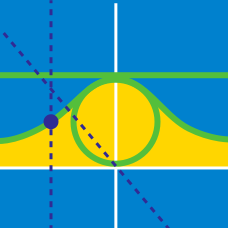Calculus

# Parametric Equations - Surface Area

What is the surface area $$S$$ of the body of revolution obtained by rotating the curve $$y=e^x,$$ $$0 \le x \le 1,$$ about the $$x-$$axis?

What is the surface area $$S$$ of the body of revolution obtained by rotating the parametric curve $\begin{array} &x = 8 t^2 + 9 &y = -4 t &0 \leq t \leq 1 \end{array}$ about the $$x$$-axis?

What is the surface area $$S$$ of the solid of revolution obtained by rotating the parametric curve $\begin{array} &x = 4 t &y = 6 t &0 \leq t \leq 2 \end{array}$ about the $$y$$-axis?

What is the surface area $$S$$ of the body of revolution obtained by rotating the parametric curve $\begin{array} &x = 7 \cos t &y = 7 \sin t &0 \leq t \leq \pi \end{array}$ about the $$x$$-axis?

If $$S$$ is the surface area of the solid obtained by rotating the parametric curve $\begin{array} &x = 4 \cos^3 t &y = 4 \sin^3 t &0 \leq t \leq \frac{\pi}{2} \end{array}$ about the $$x$$-axis, what is $$\frac{5}{2}S?$$

×